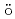We study the Pauli-Schrdinger equation in a uniformly rotating frame of reference to describe a coupling of spins and mechanical rotations. The explicit form of the spin-orbit interaction (SOI) with the inertial effects due to the mechanical rotation is presented. We derive equations of motion for a wave packet of electrons in two-dimensional planes subject to the SOI. The solution is a superposition of two cyclotron motions with different frequencies and a circular spin current is created by the mechanical rotation.